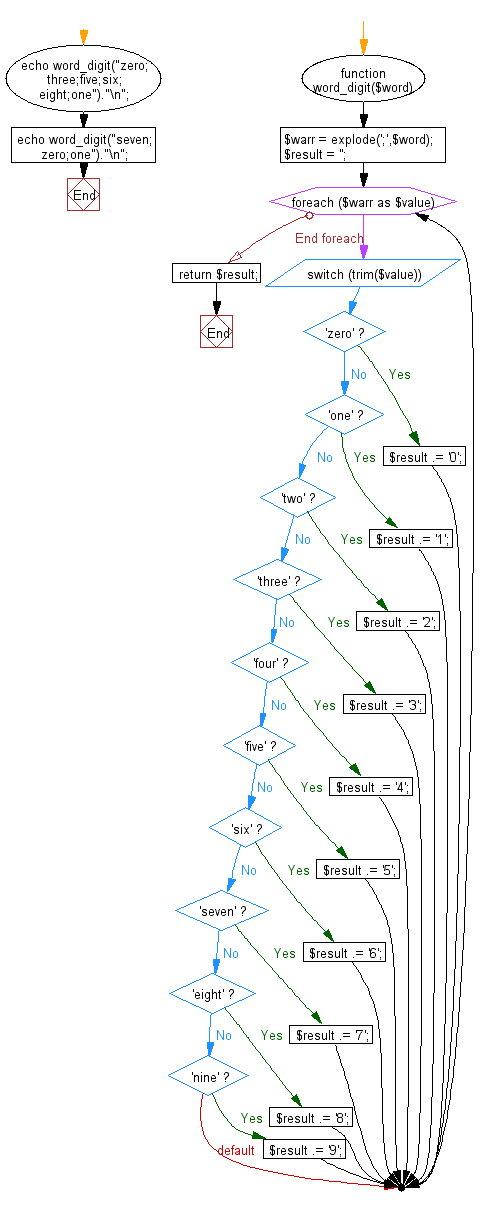﻿ PHP Exercise: Convert word to digit - w3resource

# PHP Exercises: Convert word to digit

## PHP: Exercise-33 with Solution

Write a PHP program to convert word to digit.

Input: zero;three;five;six;eight;one
Output: 035681

Sample Solution: -

PHP Code:

``````<?php
function word_digit(\$word) {
\$warr = explode(';',\$word);
\$result = '';
foreach(\$warr as \$value){
switch(trim(\$value)){
case 'zero':
\$result .= '0';
break;
case 'one':
\$result .= '1';
break;
case 'two':
\$result .= '2';
break;
case 'three':
\$result .= '3';
break;
case 'four':
\$result .= '4';
break;
case 'five':
\$result .= '5';
break;
case 'six':
\$result .= '6';
break;
case 'seven':
\$result .= '7';
break;
case 'eight':
\$result .= '8';
break;
case 'nine':
\$result .= '9';
break;
}
}
return \$result;
}

echo word_digit("zero;three;five;six;eight;one")."\n";
echo word_digit("seven;zero;one")."\n";
?>
```
```

Sample Output:

```035681
701
```

Flowchart:PHP Code Editor:

Improve this sample solution and post your code through Disqus.

What is the difficulty level of this exercise?

Test your Programming skills with w3resource's quiz.

﻿

## PHP: Tips of the Day

Returns all elements in an array except for the first one

Example:

```<?php
function tips_tail(\$items)
{
return count(\$items) > 1 ? array_slice(\$items, 1) : \$items;
}

print_r(tips_tail([1, 5, 7]));
?>
```

Output:

```Array
(
 => 5
 => 7
)
```